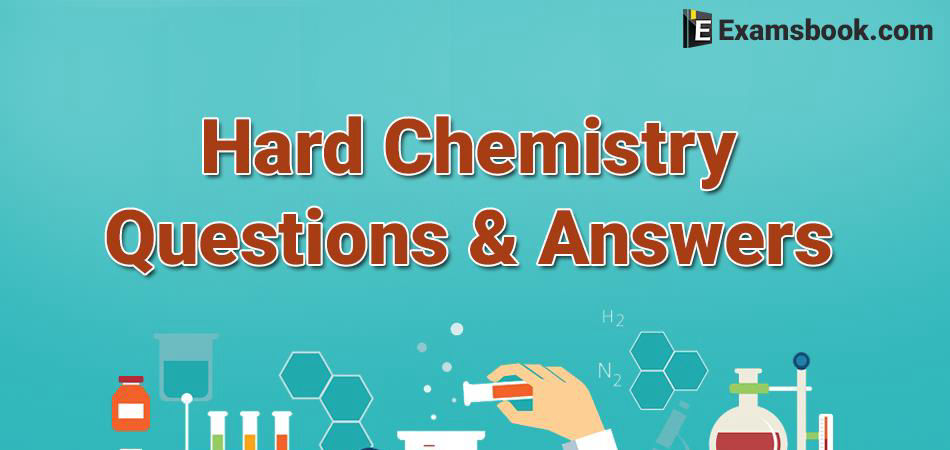• Save

# Hard Chemistry Questions and Answers for SSC ExamChemistry General Knowledge questions asked in many competitive exams and also chemistry GK is important for SSC and Banking exams. Practice with important  Hard Chemistry Questions and Answers for SSC Exam.

You should also check physics GK questions , Biology Gk Questions and General Science GK questions for better practice of competitive exams.

Here you can learn chemistry general knowledge with the collection of 40important Hard Chemistry Questions and Answers for SSC Exam. Prepare for competitive exam by practice chemistry question and answers.

## Hard Chemistry Questions and Answers

Q :  Which of the following gas is used in cigarette lighters ?

(A) Butane

(B) Propane

(C) Methane

(D) Ethane

Q :  Which of the following is produced during the formation of photochemical smog ?

(A) Nitrogen Oxide

(B) Hydrocarbons

(C) Methane

(D) Ozone

Q :  The metallurgical process in which a metal is obtained in a fused state is called ?

(A) roasting

(B) calcinations

(C) smelting

(D) froth floatation

Q :  Which gas is used in fire extinguishers ?

(A) Carbon dioxide

(B) Nitrogen oxide

(C) Carbon monoxide

(D) Sulpher dioxide

Q :  The metal used to recover copper from a solution of copper sulphate is ?

(A) Na

(B) Ag

(C) Hg

(D) Fe

Q :  Which of the primary component of natural gas?

(A) Ethane

(B) Propane

(C) Methane

(D) Butane

Q :  The oxide of Nitrogen used in medicine as anaesthetic is ?

(A) Nitrogen pentoxide

(B) Nitrous oxide

(C) Nitric oxide

(D) Nitrogen dioxide

Q :  Which one of the following metals does not react with water to produce Hydrogen?

(B) Lithium

(C) Potassium

(D) Sodium

Q :  The most electronegative element among the following is

(A) sodium

(B) bromine

(C) fluorine

(D) oxygen

Q :  Atomic number is equal to

(A) Number of electrons

(B) Numbers of protons

(C) Number of nutron

(D) Total number of protons and neutrons